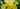# Implementing a Bloom filter - Part 3

Last time we looked at how the internals of Bloom filters work. In this post, we'll get our hands dirty and implement it.

We'll set up an interface with pure virtuals.

``````class IBloomFilter
{
public:
virtual void put(std::string input) = 0;
virtual bool isMaybePresent(std::string input) const = 0;
};``````

Now we'll need to pick something to use as our array of bits. The two obvious choices are `std::bitset` and `std::vector<bool>`. Since we want the user of the Bloom filter to specify its size at construction time, we will need to go with the vector.

Here is our class definition.

``````class BloomFilter : public IBloomFilter {

public:
BloomFilter(const Hash& hash, int size, int k);

// Inherited via IBloomFilter
virtual void put(std::string input) override;
virtual bool isMaybePresent(std::string input) const override;

private:
Hash m_hash;
int m_k;
std::vector<bool> m_vector;
};``````

Now, you might be asking how we will pick `k` hashing functions. What we actually need is `k` functions which will somehow hash the input, give us `k` different indexes into our bit vector and ensure that these results are consistent.

But, how can we pick `k` different ways of hashing? There are only a fixed number of hashing algorithms, and in theory our `k` could be anything. Luckily, there is a way of generating a different hash based on the iteration number, this technique is called double hashing. Let's see how this works.

``h(input, iteration) = h1(input) + iteration * h2(input)``

So all we have to do is pick 2 different hashing functions, and for every iteration compute the final hash using the formula above.

We will use Murmur Hash for generating the two unique hashes.

``````std::array<uint32_t, 2> Hash::hash(const std::string & input) const
{
std::array<uint32_t, 2> out;
MurmurHash3_x86_32(input.data(), sizeof(input.data()), 0, out.data());

return out;
}``````

And now we just apply the forumula for every iteration. We also modulo with the size of our filter to make sure the numbers we get are actually in our Bloom filter range.

``````std::vector<uint32_t> Hash::hash(const std::string& input, unsigned int iterations, unsigned int max) const
{
auto hashesIndexed = std::vector<uint32_t>();
auto hashedInput = this->hash(input);
auto firstHash = hashedInput;
auto secondHash = hashedInput;

for (unsigned int i = 0; i < iterations; i++)
{
auto hashedInputInt = (firstHash + secondHash * i) % max;
hashesIndexed.push_back(hashedInputInt);
}

return hashesIndexed;
}``````

Now that we have all the moving parts, we can easily implement `put` and `isMaybePresent`.

``````void BloomFilter::put(std::string input)
{
// Hash and get k indexes
auto& indexes = m_hash.hash(input, m_k, m_vector.size());

// Set all those bits to 1
for (const auto& index : indexes)
{
m_vector[index] = true;
}
}

bool BloomFilter::isMaybePresent(std::string input) const
{
// Hash and get k indexes
auto& indexes = m_hash.hash(input, m_k, m_vector.size());

// Find if all bits are set
for (const auto& index : indexes)
{
if (m_vector[index] == false)
{
return false;
}
}

return true;
}``````

And this is pretty much it. You can see the full code at iolivia/bloom-filter. Next up we'll do some experiments with `n` and `k` and see what our error rates are.